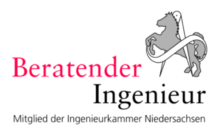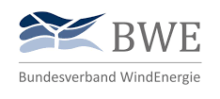## Accelerations

The Accelerations is a physical quantity and describes the change in the velocity of a body over a defined period of time. It is a directional variable and is used to describe movement processes.

The measurement and assessment of accelerations plays an important role, especially in the field of technical systems with dynamic loads. On the one hand, vibrations can be introduced into the substructure of industrial or wind turbines by operating the machines. In these cases, it must be checked to what extent this leads to premature ageing of the construction. On the other hand, certain limit values must be observed for the smooth operation of electrical installations.

By using acceleration sensors , accelerations can be measured and analyzed over a certain period of time. The company SKI has piezoelectric acceleration sensors available for this purpose, which convert the dynamic pressure fluctuations into electrical signals via sensor plates. These signals are transferred to the connected data logger and stored. By means of comparison models and natural frequency analyses of the designs, conclusions can be drawn on the effects of the accelerations.

## Piezoelectric acceleration sensors

In the piezoelectric principle, a piezoelectric material is fixed in a case. Inside the housing, two electrode surfaces face each other. If a force or an acceleration across the surfaces now acts on the housing, there is a charge shift. This displacement causes a voltage change in the electrode surfaces that can be recorded. By converting the signals so different sizes can be measured, such. Pressure, force or accelerations.

The schematic structure of a piezoelectric accelerometer is shown in the figure below. The sensor essentially consists of two components:

• Piezoelectric material
• Seismic mass

The seismic mass is coupled to the piezoelectric material that acts as a fixed abutment. If the upper mass is set into vibration, the inertia of the mass acts as a force on the disk. As the force after Newton is composed of mass times acceleration, the piezoelectric effect creates a charge which can be put into proportion. By this, the accelerations can be dissipated by the inertia of the mass and the force acting on the surfaces.

The seismic mass is coupled to the piezoelectric material that acts as a fixed abutment. If the upper mass is set into vibration, the inertia of the mass acts as a force on the disk. As the force after Newton is composed of mass times acceleration, the piezoelectric effect creates a charge which can be put into proportion. By this, the accelerations can be dissipated by the inertia of the mass and the force acting on the surfaces.

Accelerometers with IEPE technology (Integrated Electronics Piezo Electric) is a standard for piezoelectric sensors with built-in impedance converter electronics. The technology ensures that the signal of the piezoelectric material is converted into a low-impedance voltage signal that can be transmitted over the cable lengths with low loss. The sensor is supplied by a simple coaxial cable, which allows the supply voltage and the sensor signal to be transmitted via one cable. Thus expensive low noise cables can be avoided. The sensors are operated with constant current, which is in the range of 2 to 20 mA. The interference sensitivity and the output impedance increase as the supply current decreases.

Therefore most sensors are supplied with 4 mA, as this is a good ratio between noise immunity and current consumption. The working voltage of the measuring signal is superimposed as an alternating voltage (8 to 12 V) on the working voltage and must then be filtered out by the data logger.

## Services

SKI Ingenieurges. mbH offers the following services in the field of acceleration measurements:

• Test accompanying and monitoring activities
• Construction and calibration of numerical models for recalculation
• Recalculation of test results in the static, dynamic and transient range
• Evaluation and interpretation of measurement results, as well as comparison of numerically supported test methods
• Creation of measurement concepts, reports and expert opinionsSKI Ingenieurges. mbH
Mengendamm 12
30177 Hannover

Phone: +49 (0) 511 / 261847-0

## Consulting Engineers